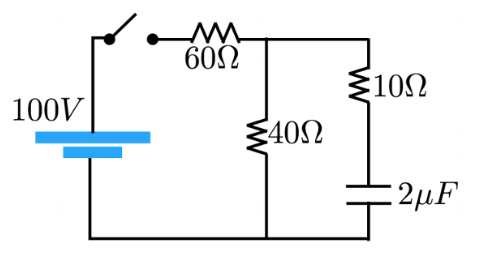# Problem: RC Circuit Analysis After the switch has been closed a very long time, what is the current through the 10Ω? resistor?

###### FREE Expert Solution

The charging current for a capacitor:

$\overline{){\mathbf{I}}{\mathbf{=}}\frac{{\mathbf{V}}_{\mathbf{b}\mathbf{a}\mathbf{t}\mathbf{t}\mathbf{e}\mathbf{r}\mathbf{y}}}{\mathbf{R}}{{\mathbf{e}}}^{\mathbf{-}\mathbf{\left(}\mathbf{t}\mathbf{/}\mathbf{R}\mathbf{C}\mathbf{\right)}}}$

After a very long time, e-(t/RC) approaches e-∞.

96% (44 ratings)###### Problem Details

RC Circuit Analysis

After the switch has been closed a very long time, what is the current through the 10Ω? resistor?Frequently Asked Questions

What scientific concept do you need to know in order to solve this problem?

Our tutors have indicated that to solve this problem you will need to apply the !! Resistor-Capacitor Circuits concept. If you need more !! Resistor-Capacitor Circuits practice, you can also practice !! Resistor-Capacitor Circuits practice problems.